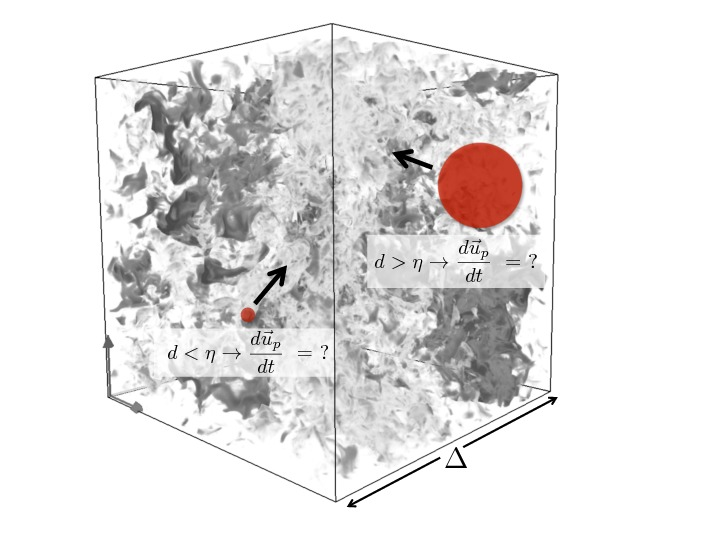# Laboratoire de Mécanique des Fluides et d’Acoustique - UMR 5509

LMFA - UMR 5509
Laboratoire de Mécanique des Fluides et d’Acoustique
Lyon
France

Rémi Zamanski - Institut de Mécanique des Fluides de Toulouse

## Stochastic model for the particle dynamics in LES of turbulent flows

Vendredi 20 janvier 2017 - 11h - Bât H10 salle B11 - ECL

In the context of Large Eddy Simulation (LES) of turbulent flows laden with solid particles, we propose a model to account for the unresolved scales of the flow in the particles dynamics. The small scales of a high Reynolds number flow are characterized by strong velocity gradients. To account for those gradients, and specifically the turbulent motion at scales shorter/smaller than the particles characteristics scales, we decompose the particle acceleration in its resolved and residual parts. In the latter, the interactions with the inertial range of the turbulent flow are simulated by a stochastic process evolving along the particle trajectory. We consider both particles much smaller and larger than the Kolmogorov length scale. In the case of the small particles, we introduced two processes, one for its norm, and another for its direction. Results showed that by introducing the stochastic model for the particle residual acceleration, the particle acceleration statistics from DNS is predicted fairly well. For the particles bigger than the Kolmogorov scale, we propose another stochastic model. We derived a fluctuating drag, simulated by lognormal process. This model gives stretched tails in the particle acceleration distribution and dependence of its variance in agreement with experiments.Contact LMFA : Wouter Bos